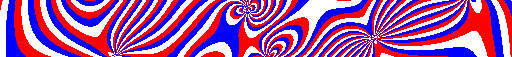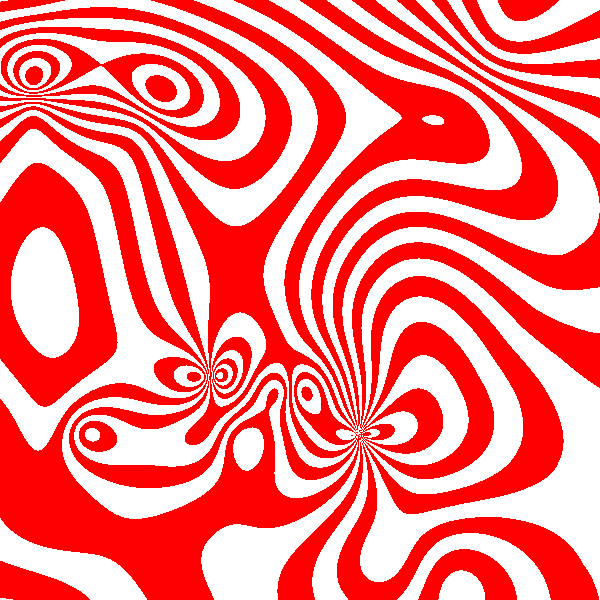Posted on December 5, 2018

So I thought it’d make a cool graphical effect to take a cube with some electrical charges inside and then make an image for each slice through this cube, converting each point in the slice to a pixel colour based on some function. In this case, I used a modulo function to get some nice stripes but I imagine a lot of other cool effects could be made in a similar way.

First, we’ll need a way to calculate the field strength at a given point. This is done using the electrical field strength formula:

$E = \frac{1}{4\pi\epsilon_0} \frac{Q}{r^2}$

If you’ve ever done a physics A-level you’ll recognise this immediately, but what it’s basically saying is that the field strength is proportional to the charge of the object, and inversely proportional to the square of the distance away from it. The complicated looking $$\frac{1}{4\pi\epsilon_0}$$ is an “arbitrary” constant to do with circles and stuff, but we can set this to whatever we like purely to make the maths work out more nicely.

Problem is, this is only for one point, and only calculates the magnitude of the field at that point. The magnitude is what we want in the end, but when we start adding up multiple charges we need the field strengths to be vectors so, for example, two charges could cancel each other out.

To this end, we can generalise the formula to this one, taking into account directions and vectors and all that.

$E_{total}(\rho) = \sum_\xi{(\frac{|\xi - \rho|}{4\pi\epsilon_0} \frac{Q_\xi}{||\xi - \rho||^2})}$

$$\rho$$ is the position, because it kind of looks like “p” but is more fancy. This is the variable that will be changed for each pixel in a slice. And, $$\xi$$ is the position of the charge - that’s the variable that the for-loop, uh.. summation, ranges over. This formula is basically just the other one, but multiplied by the direction vector and replacing $$r$$ for an actual calculation of the distance.

So now we have a function to get the field strength at each position. Well, it gets the field vector, but the scalar strength is simply $$||E_{total}||$$. All that’s left is to write a function to calculate the colour for a given field strength. I’ll do that with this, for now:

$Colour(E) = \left\{ \begin{array}{ll} red & \lfloor E \rfloor \mod 2 = 0 \\ white & otherwise \\ \end{array} \right.$

Ok, that wasn’t quite the last thing. I mean, it would work at this point, but the constant of proportionality $$\frac{1}{4\pi\epsilon_0}$$ is $$8987551788$$ which is massive so all the colours will be mushed together. It’s an easy fix, though, I’ll just redefine $$\frac{1}{4\pi\epsilon_0} = 10000$$ which seems to work well enough for this. The actual best value to use here will be based on the resolution you decide to render at.

Last thing now is to write a program to render this into a nice animation for me. I’ve done that for you, here.

It’s difficult to render an entire 3D image to a 2D monitor$$^{[citation\ needed]}$$, hence the animations - note this isn’t animating through time, though, the animation is through the z-axis.